JEE  >  Sample JEE Main Physics Mock Test

# Sample JEE Main Physics Mock Test

Test Description

## 25 Questions MCQ Test | Sample JEE Main Physics Mock Test

Sample JEE Main Physics Mock Test for JEE 2023 is part of JEE preparation. The Sample JEE Main Physics Mock Test questions and answers have been prepared according to the JEE exam syllabus.The Sample JEE Main Physics Mock Test MCQs are made for JEE 2023 Exam. Find important definitions, questions, notes, meanings, examples, exercises, MCQs and online tests for Sample JEE Main Physics Mock Test below.
Solutions of Sample JEE Main Physics Mock Test questions in English are available as part of our course for JEE & Sample JEE Main Physics Mock Test solutions in Hindi for JEE course. Download more important topics, notes, lectures and mock test series for JEE Exam by signing up for free. Attempt Sample JEE Main Physics Mock Test | 25 questions in 60 minutes | Mock test for JEE preparation | Free important questions MCQ to study for JEE Exam | Download free PDF with solutions
 1 Crore+ students have signed up on EduRev. Have you?
Sample JEE Main Physics Mock Test - Question 1

### Number of electrons emitted by a surface exposed to light is directly proportional to

Detailed Solution for Sample JEE Main Physics Mock Test - Question 1

Key Idea Number of photoelectrons emitted proportional to intensity of incident light.

For a given metal, rate of emission of photo electrons i.e., photocurrent is directly proportional to the intensity of incident radiation for a given light i.e., a bright light always gives more photocurrent than a dim one for a given frequency.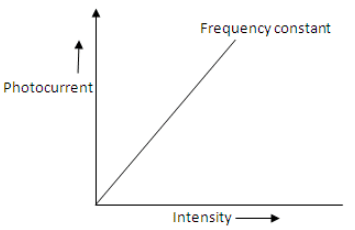Intensity here means energy incident normally per unit area per second and is also called, energy flux or power density.

Sample JEE Main Physics Mock Test - Question 2

### In photoelectric emission the number of electrons ejected per second

Detailed Solution for Sample JEE Main Physics Mock Test - Question 2

For a fixed frequency of incident radiation, the number of electron ejected per second increases linearly with increase in intensity of incident light.

Sample JEE Main Physics Mock Test - Question 3

### A travelling microscope is held vertical and focussed at a point object 'O'. The reading of the microscope is 9.65 cm. A thick glass slab is placed on O and its image O’ was observed with the microscope by refocusing it. The reading was now 11.65 cm. Finally a few particles of lycopodium powder is sprinkled on the top of the glass slab and it is viewed with the microscope by re-focusing. The reading of microscope is found to be 12.85 cm. The refractive index of the material of the glass slab is

Sample JEE Main Physics Mock Test - Question 4

According to Hooke's law of elasticity, if stress is increased, the ratio of stress to strain

Detailed Solution for Sample JEE Main Physics Mock Test - Question 4

Young's modulus (Y), the ratio of stress to strain ,remains constant for a particular material. i.e.
Y= stress/ strain=constant

Sample JEE Main Physics Mock Test - Question 5

A step-up transformer operates on a 230 V line and supplies a load of 2 ampere. The ratio of the primary and secondary windings is 1 : 25. The current in the primary is

Detailed Solution for Sample JEE Main Physics Mock Test - Question 5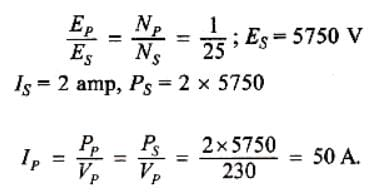Sample JEE Main Physics Mock Test - Question 6

If the distance between the plates of parallel plate capacitor is halved and the dielectric constant of dielectric is doubled, then its capacity will

Detailed Solution for Sample JEE Main Physics Mock Test - Question 6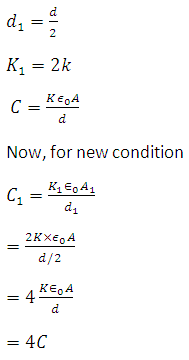Sample JEE Main Physics Mock Test - Question 7

Two thin wire rings each having a radius R are placed at a distance d apart with their axes coinciding. The charges on the two rings are +q and -q. The potential difference between the centres of the two rings is

Detailed Solution for Sample JEE Main Physics Mock Test - Question 7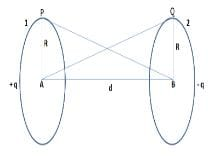Figure shows the two ring 1 and 2 each of radius R and having charges +q and −q
Let A and B be the centers of the two rings and VA and VB be the respective potentials at these points, then
VA= Potential due to ring 1+ Potential due to ring 2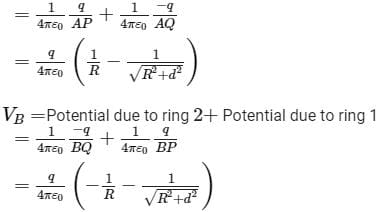Therefore potential difference between the two rings,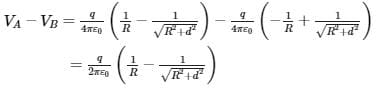Sample JEE Main Physics Mock Test - Question 8

A mass of a gas exerts a pressure of 72 cm of mercury at 27o cm of mercury at 27oC. It is heated at constant volume and the pressure after some time becomes 90 cm of mercury. What is the temperature of the gas?

Detailed Solution for Sample JEE Main Physics Mock Test - Question 8

(P1/T1)=(P2/T2)
T2=(P2.T1)/P1
T2=(90x300)/72
T2=375 K
T2=375-273 = 102oC

Sample JEE Main Physics Mock Test - Question 9

The mass of the earth is 81 times that of the moon and the radius of earth is 3.5 times that of the moon. The ratio of the acceleration due to gravity at the surface of the moon to that at the surface of the earth is

Detailed Solution for Sample JEE Main Physics Mock Test - Question 9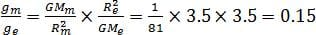Sample JEE Main Physics Mock Test - Question 10

A particle moves in a circular orbit under the action of a central attractive force inversely proportional to the distance 'r'. The speed of the particle is

Detailed Solution for Sample JEE Main Physics Mock Test - Question 10

We know that the centripetal force F = k/r
For some constant k and radius of curvature r.
Also we know centripetal force F = mv2 /r
Thus we get v = √k/m.

Sample JEE Main Physics Mock Test - Question 11

A nucleus with Z = 92 emits the following in sequence: α , α , β , β , α , α , α , α ; β , β , α , β+ , β+ , α . The Z of the resulting nucleus is

Detailed Solution for Sample JEE Main Physics Mock Test - Question 11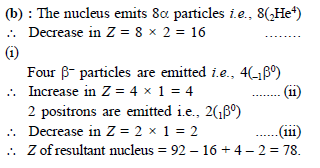Sample JEE Main Physics Mock Test - Question 12

In the following question, a Statement of Assertion (A) is given followed by a corresponding Reason (R) just below it. Read the Statements carefully and mark the correct answer-
Assertion(A): Virtual images are always erect.
Reason (R): Virtual images are formed by diverging lenses only.

Detailed Solution for Sample JEE Main Physics Mock Test - Question 12

The virtual image is always erect. The common example of virtual image is the image formed in the mirror when we stand in front of that mirror. A real image is that image which is formed when the light rays coming from an object actually meet each other after reflection or refraction.

Sample JEE Main Physics Mock Test - Question 13

In the following question, a Statement of Assertion (A) is given followed by a corresponding Reason (R) just below it. Read the Statements carefully and mark the correct answer-
Assertion(A): According to law of conservation of mechanical energy sum of kinetic energy and potential energy is always constant.
Reason(R): Mechanical energy is conserve.

Detailed Solution for Sample JEE Main Physics Mock Test - Question 13

Conservation of Mechanical Energy

The sum total of an object’s kinetic and potential energy at any given point in time is its total mechanical energy.  The law of conservation of energy says “Energy can neither be created nor be destroyed.”

So, it means, that, under a conservative force, the sum total of an object’s kinetic and potential energies remains constant.

Sample JEE Main Physics Mock Test - Question 14

When seen in green light, the saffron and green portions of our National Flag will appear to be

Detailed Solution for Sample JEE Main Physics Mock Test - Question 14

When seen in green light, the saffron and green portion of our national Flag will appear to be black and green respectively, because saffron absorbs the green light completely without any reflection so is shown as black, but Green portion of the Indian national flag reflects the same green light which falls on it so it appear as green in colour only.

Sample JEE Main Physics Mock Test - Question 15

Which is not true; velocity of sound is

Detailed Solution for Sample JEE Main Physics Mock Test - Question 15

The density of dry air is more than the moist air. When moisture is removed from air, its density increases. The speed of sound in a medium is inversely proportional to the square root of its density. Therefore, the speed of sound in moist air is more than that in dry air. Hence, correct answer is (D).

Sample JEE Main Physics Mock Test - Question 16

Heat capacity of a substance is infinite. It means

Detailed Solution for Sample JEE Main Physics Mock Test - Question 16

Specific heat is defined as amount of joules to make 1 gram of a substance to change the temperature by 1 celsius; if the specific heat is infinite, that means it takes infinite amount of joules to change temperature, thus no change in temperature takes place even if you add or subtract heat because it would take infinite energy to change the temperature.

Sample JEE Main Physics Mock Test - Question 17

Two tuning forks A and B produce 6 beats per second when sounded together. When B is slightly loaded with wax, the beats are reduced to 4 per second. If the frequency of A is 512 Hz, the frequency of 'B' is

Detailed Solution for Sample JEE Main Physics Mock Test - Question 17

When loaded with wax, frequency of beats is reduced.
⇒ Frequency of B is higher than that of A.
⇒fB​−fA​=6
⇒fB​=(512+6)=518 Hz

Sample JEE Main Physics Mock Test - Question 18

The K.E. of a light and a heavy object is same Which object has more momentum?

Sample JEE Main Physics Mock Test - Question 19

In Young's double slit experiment, an interference pattern is obtained on a screen by a light of wavelength 6000 Å coming from the coherent sources S1 and S2. At certain point P on the screen third dark fringe is formed. Then the path difference S1p − S2p in microns is

Detailed Solution for Sample JEE Main Physics Mock Test - Question 19

For dark fringe at P  S1p − S2p =Δ=(2n−1)λ/2
Here n =3 and l = 6000
So,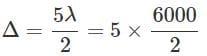=15000AA = 1.5 micron

Sample JEE Main Physics Mock Test - Question 20

Two rods 1 and 2 are released from rest as shown in figure.
Given: l1=4l, m1=2m, l= 2l and m= ml1=4l, m1=2m,l2=2l and m2=m. There is no friction between the two rods. If αα be the angular acceleration of rod 1 just after the rods are released. Then :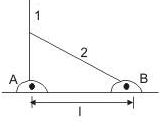Q. What is the normal reaction between the two rods at this instant ?

*Answer can only contain numeric values
Sample JEE Main Physics Mock Test - Question 21

At what speed a ball must be projected vertically upward so that distance travelled by it in 5th second is equal to distance travelled in sixth second (in m/s):-

Detailed Solution for Sample JEE Main Physics Mock Test - Question 21

time of flight = (5 sec) × 2 = 10 sec
µ = gt = 9.8 × 5 = 49 m/s

*Answer can only contain numeric values
Sample JEE Main Physics Mock Test - Question 22

In thermodynamic process pressure of a fixed mass of gas is changed in such a manner that the gas releases 30 joule of heat and 18 joule of work was done on the gas. If the initial internal energy of the gas was 60 joule, then, the final internal energy (in J) will be:

Detailed Solution for Sample JEE Main Physics Mock Test - Question 22

ΔQ = –30J
Δw = –18
Ui = 60 J
Uf = ?
ΔQ = Δw + Uf – Ui
–30 = –18 + Uf – 60 ⇒ Uf = 48 J

*Answer can only contain numeric values
Sample JEE Main Physics Mock Test - Question 23

In a certain amplitude modulate wave, maximum amplitude is 8V. If modulation factor is 60% then minimum amplitude of the wave, will be-

Detailed Solution for Sample JEE Main Physics Mock Test - Question 23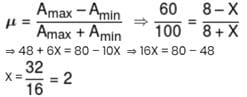*Answer can only contain numeric values
Sample JEE Main Physics Mock Test - Question 24

Figure shows a Meter bridge wire AC has uniform cross-section. The length of wire AC is 100 cm. X is a standard resistor of 4Ω and Y is a coil. When Y is immersed in melting ice the null point is at 40 cm from point A. When the coil Y is heated to 100ºC, a 12Ω resistor has to be connected in parallel with Y in order to keep the bridge balanced at the same point. The temperature coefficient of resistance of the coil is x × 10–2 SI units. Find the value of x.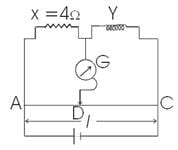Detailed Solution for Sample JEE Main Physics Mock Test - Question 24

This is linear variation of resistance.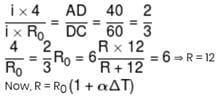12 = 6 (1 + α 100)
α = 1 × 10–2
x = 1

*Answer can only contain numeric values
Sample JEE Main Physics Mock Test - Question 25

Two cars moving in opposite directions approach each other with speed of 22 m/s and 16.5 m/s respectively. The driver of the first car blows a horn having a frequency 400 Hz. The frequency heard by the driver of the second car is [velocity of sound 340 m/s] (in Hz):-

Detailed Solution for Sample JEE Main Physics Mock Test - Question 25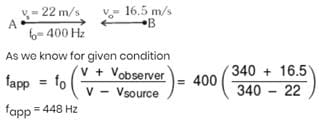Use Code STAYHOME200 and get INR 200 additional OFF Use Coupon Code
Information about Sample JEE Main Physics Mock Test Page
In this test you can find the Exam questions for Sample JEE Main Physics Mock Test solved & explained in the simplest way possible. Besides giving Questions and answers for Sample JEE Main Physics Mock Test, EduRev gives you an ample number of Online tests for practice